### RADIANS Function – Degrees to Radians – Excel, VBA, G Sheets

This Tutorial demonstrates how to use the Excel RADIANS Function in Excel to convert an angle to radians.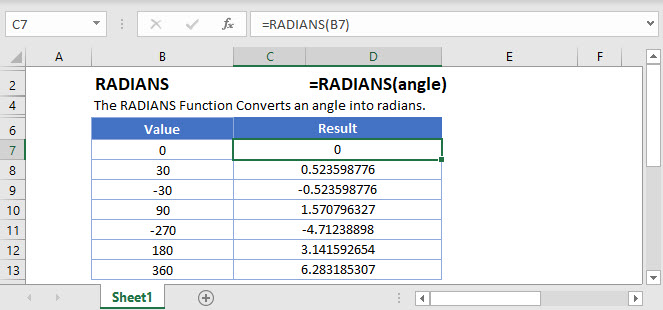## RADIANS Function Overview

The RADIANS Function Converts an angle into radians.

To use the RADIANS  Excel Worksheet Function, select a cell and type: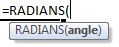(Notice how the formula inputs appear)

## RADIANS Function Syntax and Inputs:

angle – An angle in degrees.

AutoMacro - VBA Code Generator

## RADIANS Function

The DEGREES Function converts radians into degrees.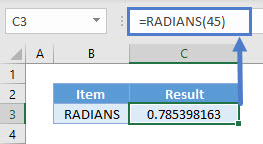## RADIANS & PI Function

The RADIANS Function takes the argument 180 &  returns the same result as PI()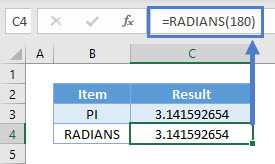## RADIANS Function – Negative Angle

The RADIANS Function can also convert negative angles into radians.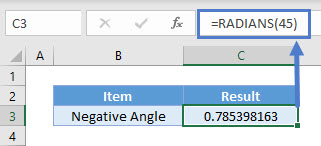## RADIANS in Google Sheets

The RADIANS Function works exactly the same in Google Sheets as in Excel: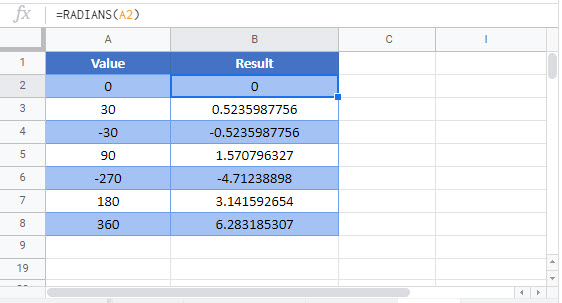## Additional Notes

Use the RADIANS Function to convert an angle into radians.

## RADIANS Examples in VBA

You can also use the RADIANS function in VBA. Type:
`application.worksheetfunction.radians(angle)`

Executing the following VBA statements

will produce the following results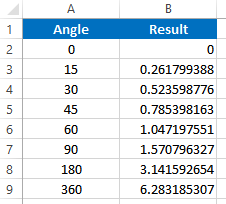For the function arguments (angle, etc.), you can either enter them directly into the function, or define variables to use instead.

Return to the List of all Functions in Excel

## Excel Practice Worksheet

Practice Excel functions and formulas with our 100% free practice worksheets!

• Automatically Graded Exercises
• Learn Excel, Inside Excel!